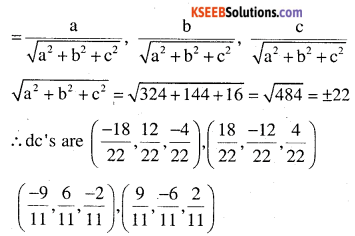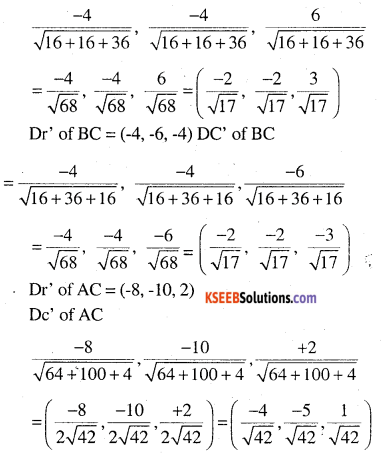# 2nd PUC Maths Question Bank Chapter 11 Three Dimensional Geometry Ex 11.1

Students can Download Maths Chapter 11 Three Dimensional Geometry Ex 11.1 Questions and Answers, Notes Pdf, 2nd PUC Maths Question Bank with Answers helps you to revise the complete Karnataka State Board Syllabus and score more marks in your examinations.

## Karnataka 2nd PUC Maths Question Bank Chapter 11 Three Dimensional Geometry Ex 11.1

### 2nd PUC Maths Three Dimensional Geometry NCERT Text Book Questions and Answers Ex 11.1

Question 1.
If a line makes angles 90°, 135°, 45° with the x, y and z-axes respectively, find its direction cosines.
Dc’s are cos α, cos β and cos γ
cos 90°, cos 135°, cos 45°
$$\left(0, \frac{-1}{\sqrt{2}}, \frac{1}{\sqrt{2}}\right)$$

Question 2.
Find the direction cosines of a line which makes equal angles with the coordinate axes.
Let the angle be α,α,α
Direction cosines are cos α, cos α , cos α
but cos2 α + cos2 β2 + cos2 γ = 1
cos2 α + cos2 α+ cos2 α = 1 ⇒ 3cos2 α= 1Question 3.
If a line has the direction ratios -18, 12, – 4, then what are its direction cosines ?
a : b : c = -18 : 12 : -4
let l1, m1, n are the dc’sQuestion 4.
Show that the points (2, 3, 4), (- 1, – 2, 1), (5, 8, 7) are collinear.
Let A (2, 3, 4), B (-1, -2, 1) C (5, 8, 7)
Directions of AB = (-1 -2, -2 -3, +1, – 4) =(-3, -5, -3)
Dr’s of AC = (5-2, 8-3, 7-4) = (3, 5, 3)
Dr’ of AB and AC are proportional.
Hence AB and AC are parallel. But A is common
∴ points A, B, C are collinearQuestion 5.
Find the direction cosines of the sides of the triangle whose vertices are
(3, 5, – 4), (- 1, 1, 2) and (- 5, – 5, – 2).